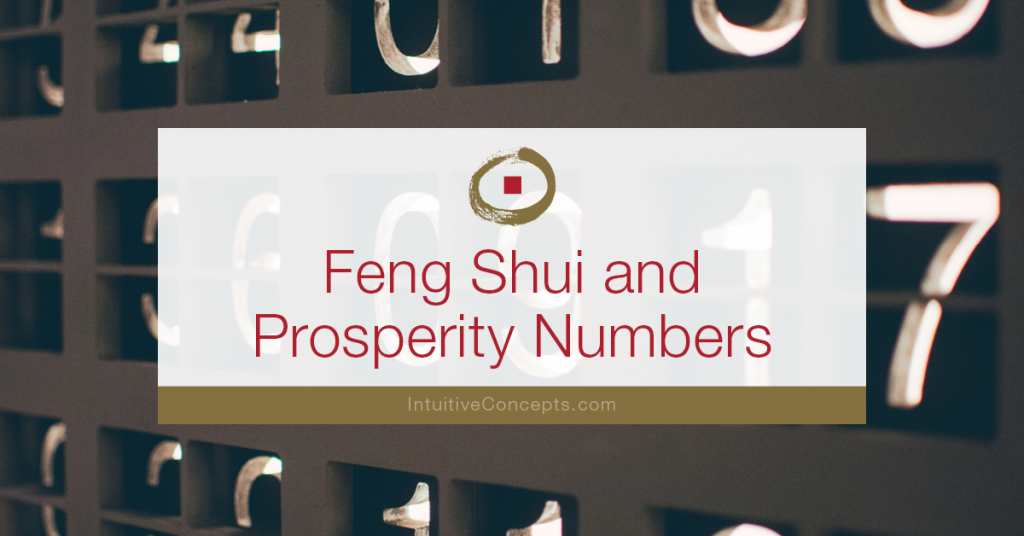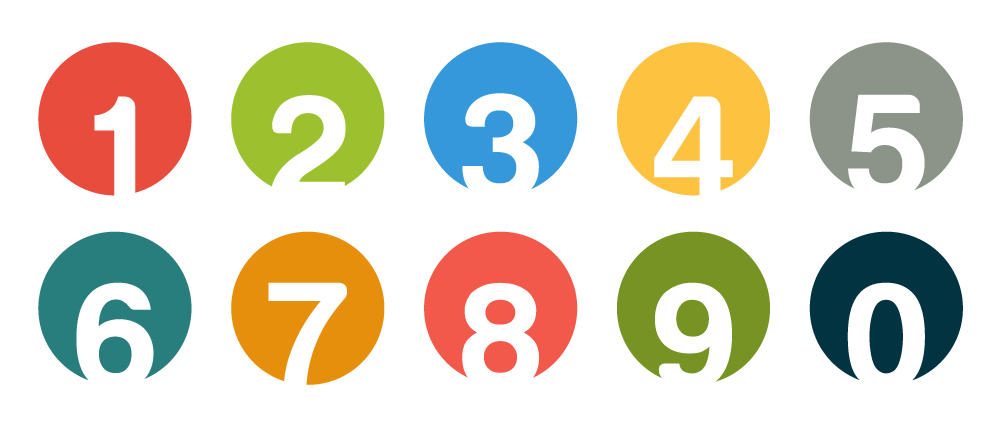# Have you heard the cliché money attracts money?

Are there disciplines the wealthy use so their money makes more money? Sure there are. Whether it’s utilizing financial planners, maintaining a balance between income and debt, or saving little on a recurring basis…there is credence in the abundance conversation.

## It’s self-talk…what we say to ourselves.

Let me explain.

If on a daily basis we acknowledge our life as a whole, loving, and complete (we have what we need), then we attract more of that energy.

If, on the other hand, we acknowledge life as less full than it can be, we attract scarcity. Our choice creates our perception! This isn’t to say we need to wear rose-colored glasses all the time; that life doesn’t have its ups and downs. It’s about how we choose to view our experiences overall.

### I’d like to share some advice on using prosperity numbers that my commercial and residential clients use to raise their prosperity energy because it works and it’s fun.

Commercial clients, as an example, use this information to change their prices. Residential clients use it for budgets and even to request telephone numbers.

## There are numbers that improve luck, and those that deplete it.## Prosperity numbers are 6 and 8.

### The first approach is to use numbers at Face Value (examples are 16, 18, 68, 69, 86, or 89).

Another approach is for the series of numbers to total 6 or 8. Examples are 15 (1+5 = 6) or 17 (1+7 =8)–as you can see, these are reduced to a single digit by totaling the numbers. It’s particularly powerful when there are several Face-Value numbers that total to 6 or 8. For example, the phone number 888-689-6891 is an 8.

The phone number is calculated like this: 8+8+8+6+8+9+6+8+9+1 =71. Then 7+1=8.

## How can you raise your prosperity energy?

If you need to make a payment for \$14, make it for \$15 instead. If you’re selling your house, instead of \$158,000, ask for \$161,000. Use the chart below as a guideline.

Remember, numbers that show a prosperous Face Value AND a total of 6 or 8 are particularly good.

Try to avoid 2 and 5 where possible, especially next to each other (25 or 52).

 Number Equals Single Digit Face Value (FV) Avoid 6 = 6 6 6 FV 8 = 8 8 8 FV 9 = 9 9 9 FV 16 = 1 + 6 7 7 FV 18 = 1 + 8 9 9 FV 19 = 1 + 9 10 1 FV 33 = 3 + 3 6 6 36 = 3 + 6 9 9 44 = 4 + 4 8 8 60 = 6 + 0 6 6 FV 61 = 6 + 1 7 7 FV 63 = 6 + 3 9 9 68 = 6 + 8 14 5 Avoid 69 = 6 + 9 15 6 FV 71 = 7 + 1 8 8 80 = 8 + 0 8 8 FV 81 = 8 + 1 9 9 FV 86 = 8 + 6 14 5 FV Avoid 88 = 8 + 8 16 7 89 = 8 + 9 17 8 FV 90 = 9 + 0 9 9 FV 91 = 9 + 1 10 1 96 = 9 + 6 15 6 FV 98 = 9 + 8 17 8 FV 99 = 9 + 9 18 9 FV

## But if you put action behind the thought (intent), you’ll get better results!

Envision a realistic goal (reduce debt which increases income).

Be present to what you’re doing (write a check that has good energy) and don’t spend more than you can afford. Reap the reward (sense of accomplishment), and pass it along (be more generous).

May abundance be yours!